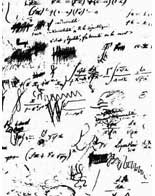Last time we have seen that in order to classify all
non-commutative $l$-points one needs to control the finite
dimensional simple algebras having as their center a finite
dimensional field-extension of $l$. We have seen that the equivalence
classes of simple algebras with the same center $L$ form an Abelian
group, the
Brauer group. The calculation of Brauer groups
is best done using
Galois-cohomology. As an aside :
Evariste Galois was one of the more tragic figures in the history of
mathematics, he died at the age of 20 as a result of a duel. There is
a whole site the Evariste Galois archive dedicated to his
work.

But let us return to a simple algebra $T$ over the
field $L$ which we have seen to be of the form $M(k,S)$, full
matrices over a division algebra $S$. We know that the dimension of
$S$ over $L$ is a square, say $n^2$, and it can be shown that all
maximal commutative subfields of $S$ have dimension n over $L$.
In this way one can view a simple algebra as a bag containing all
sorts of degree n extensions of its center. All these maximal
subfields are also splitting fields for $S$, meaning that
if you tensor $S$ with one of them, say $M$, one obtains full nxn
matrices $M(n,M)$. Among this collection there is at least one
separable field but for a long time it was an open question
whether the collection of all maximal commutative subfields also
contains a Galois-extension of $L$. If this is the case, then
one could describe the division algebra $S$ as a crossed
product
. It was known for some time that there is always a simple
algebra $S’$ equivalent to $S$ which is a crossed product (usually
corresponding to a different number n’), that is, all elements of
the Brauer group can be represented by crossed products. It came as a
surprise when S.A. Amitsur in 1972 came up with examples of
non-crossed product division algebras, that is, division algebras $D$
such that none of its maximal commutative subfields is a Galois
extension of the center. His examples were generic
division algebras
$D(n)$. To define $D(n)$ take two generic
nxn matrices
, that is, nxn matrices A and B such that all its
entries are algebraically independent over $L$ and consider the
$L$-subalgebra generated by A and B in the full nxn matrixring over the
field $F$ generated by all entries of A and B. Somewhat surprisingly,
one can show that this subalgebra is a domain and inverting all its
central elements (which, again, is somewhat of a surprise that
there are lots of them apart from elements of $L$, the so called
central polynomials) one obtains the division algebra $D(n)$ with
center $F(n)$ which has trancendence degree n^2 1 over $L$. By the
way, it is still unknown (apart from some low n cases) whether $F(n)$
is purely trancendental over $L$. Now, utilising the generic
nature of $D(n)$, Amitsur was able to prove that when $L=Q$, the
field of rational numbers, $D(n)$ cannot be a crossed product unless
$n=2^s p_1…p_k$ with the p_i prime numbers and s at most 2. So, for
example $D(8)$ is not a crossed product.

One can then
ask whether any division algebra $S$, of dimension n^2 over $L$, is a
crossed whenever n is squarefree. Even teh simplest case, when n is a
prime number is not known unless p=2 or 3. This shows how little we do
know about finite dimensional division algebras : nobody knows
whether a division algebra of dimension 25 contains a maximal
cyclic subfield (the main problem in deciding this type of
problems is that we know so few methods to construct division
algebras; either they are constructed quite explicitly as a crossed
product or otherwise they are constructed by some generic construction
but then it is very hard to make explicit calculations with
them).

Published in featured